## Logistic growth model

This vignette shows how to model general non-linear state space models with bssm. The general non-linear Gaussian model in bssm has following form:

$y_t = Z(t, \alpha_t, \theta) + H(t, \alpha_t, \theta)\epsilon_t,\\ \alpha_{t+1} = T(t, \alpha_t, \theta) + R(t, \alpha_t, \theta)\eta_t,\\ \alpha_1 \sim N(a_1(\theta), P_1(\theta)),$ with $$t=1,\ldots, n$$, $$\epsilon_t ~ N(0,\textrm{I}_p)$$, and $$\eta ~ N(0,\textrm{I}_k)$$. Here vector $$\theta$$ contains the unknown model parameters. Functions $$T(\cdot)$$, $$H(\cdot)$$, $$T(\cdot)$$, $$R(\cdot)$$,$$a_1(\cdot)$$, $$P_1(\cdot)$$, as well as functions defining the Jacobians of $$Z(\cdot)$$ and $$T(\cdot)$$ and the prior distribution for $$\theta$$ must be defined by user as a external pointers to C++ functions, which can sound intimidating at first, but is actually pretty simple, as this vignette illustrates. All of these functions can also depend on some known parameters, defined as known_params (vector) and known_tv_params (matrix) arguments to nlg_ssm functions.

As an example, consider a noisy logistic growth model \begin{aligned} y_t &= p_t + \sigma_y\epsilon, &\quad \epsilon_t \sim N(0, 1),\\ r_{t+1} &= r_t + \sigma_r\eta, &\quad \eta_t \sim N(0, 1),\\ p_{t+1} &= K p_0 \exp(t r_t) / (K + p_0 (\exp(t r_t) - 1)) + \sigma_p\xi, &\quad \xi_t \sim N(0, 1), \end{aligned}

Let’s first simulate some data, with $$\sigma_r=\sigma_p=0$$:

set.seed(1)

#parameters
K <- 100 # carrying capacity
p0 <- 10 # population size at t = 0
r <- .2 # growth rate

#sample time
dT <- .1

#observation times
t <- seq(0.1, 25, dT)

# simulate true population size (=p) at the observation times
p <- K * p0 * exp(r * t) / (K + p0 * (exp(r * t) - 1))

# observations
y <- ts(p + rnorm(length(t), 0, 5))

## Model in bssm

The functions determining the model functions are given in file model_functions.cpp. Here are few pieces from the file. The first one defines the state transition function $$T(\cdot)$$:

arma::vec T_fn(const unsigned int t, const arma::vec& alpha,
const arma::vec& theta, const arma::vec& known_params,
const arma::mat& known_tv_params) {

double dT = known_params(0);
double k = known_params(1);

arma::vec alpha_new(2);
alpha_new(0) = alpha(0);
alpha_new(1) = k * alpha(1) * exp(alpha(0) * dT) /
(k + alpha(1) * (exp(alpha(0) * dT) - 1));

return alpha_new;
}

The name of this function does not matter, but it should always return Armadillo vector (arma::vec), and have same signature (ie. the order and types of the function’s parameters) should always be like above, even though some of the parameters were not used in the body of the function. For details of using Armadillo, see Armadillo documentation. After defining the appropriate model functions, the cpp file should also contain a function for creating external pointers for the aforementioned functions. Why this is needed is more technical issue, but fortunately you can just copy the function from the example file without any modifications.

After creating the file for C++ functions, you need to compile the file using Rcpp:

Rcpp::sourceCpp("nlg_ssm_template.cpp")
## Warning in normalizePath(path.expand(path), winslash, mustWork):
## path="C:/Users/jovetale/AppData/Local/Temp/RtmpAPeq8n/
## Rbuild19f835933577/bssm/vignettes/../inst/include": The system cannot find
## the file specified
pntrs <- create_xptrs()

This takes a few seconds. et’s make less than optimal initial guess for $$\theta$$, the standard deviation of observational level noise, the standard deviations of the process noises (which were zero but let’s pretend that we do not know that), and define the prior distribution for $$\alpha_1$$:

initial_theta <- c(3, 0.5, 0.5)

# dT, K, a1 and the prior variances
psi <- c(dT, 100, 0.3, 5, 4, 10)

If you have used line // [[Rcpp::export]] before the model functions, you can now test that the functions work as intended:

T_fn(0, c(100, 200), initial_theta, psi, matrix(1))
##          [,1]
## [1,] 100.0000
## [2,] 100.0023

Now the actual model object using nlg_ssm:

library("bssm")
model <- nlg_ssm(y = y, a1=pntrs$a1, P1 = pntrs$P1,
Z = pntrs$Z_fn, H = pntrs$H_fn, T = pntrs$T_fn, R = pntrs$R_fn,
Z_gn = pntrs$Z_gn, T_gn = pntrs$T_gn,
theta = initial_theta, log_prior_pdf = pntrs$log_prior_pdf, known_params = psi, known_tv_params = matrix(1), n_states = 2, n_etas = 2) Let’s first run Extended Kalman filter and smoother using our initial guess for $$\theta$$: out_filter <- ekf(model) out_smoother <- ekf_smoother(model) ts.plot(cbind(y, out_filter$att[, 2], out_smoother$alphahat[, 2]), col = 1:3)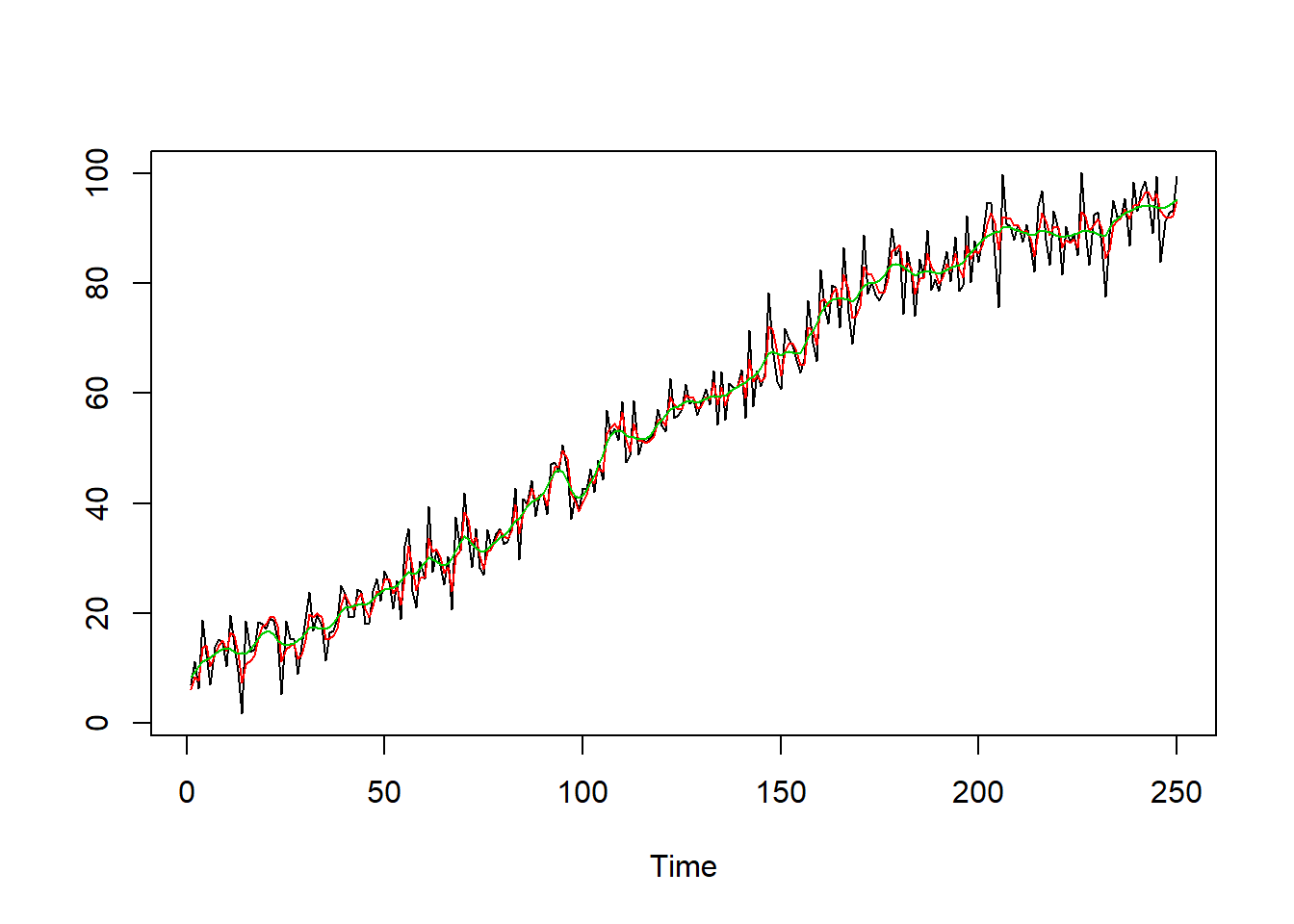ts.plot(cbind(out_filter$att[, 1], out_smoother$alphahat[, 1]), col = 1:2)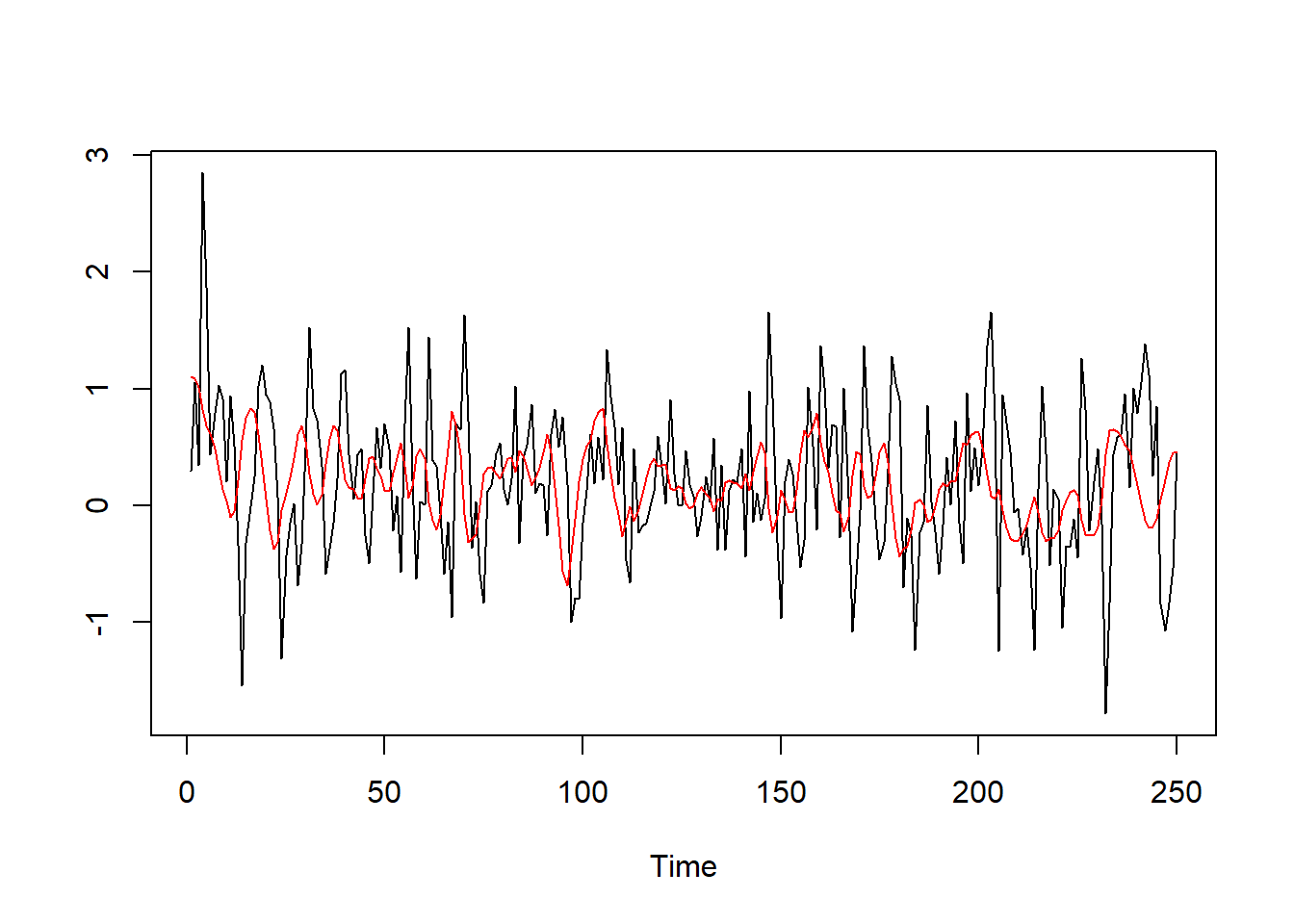## Markov chain Monte Carlo For parameter inference, we can perform full Bayesian inference with . There are multiple choices for the MCMC algorithm in the package, and here we will use $$\psi$$-PF based pseudo-marginal MCMC with delayed acceptance (note that in case of multimodal state density, it is typically better to use bootstrap filter). Let us compare this approach with EKF-based approximate MCMC (note that the number of MCMC iterations is rather small): out_mcmc_pm <- run_mcmc(model, n_iter = 5000, nsim_states = 10, method = "da", simulation_method = "psi") out_mcmc_ekf <- run_mcmc(model, n_iter = 5000, method = "ekf") summary_pm <- summary(out_mcmc_pm) summary_ekf <- summary(out_mcmc_ekf) ts.plot(cbind(summary_pm$states$Mean[, 1], summary_ekf$states$Mean[, 1]), col = 1:3)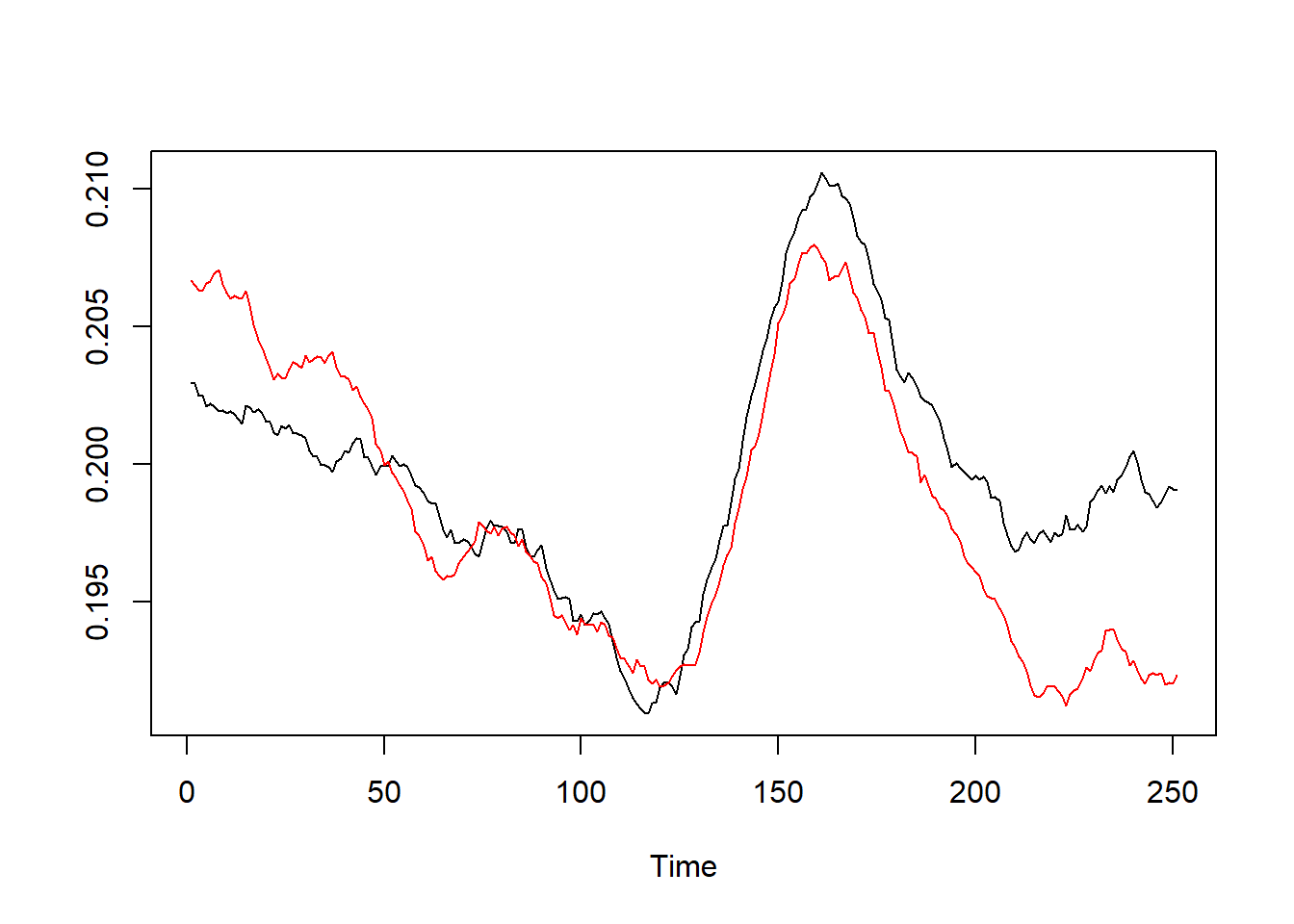ts.plot(cbind(y, summary_pm$states$Mean[, 2], summary_ekf$states$Mean[, 2]), col = 1:3)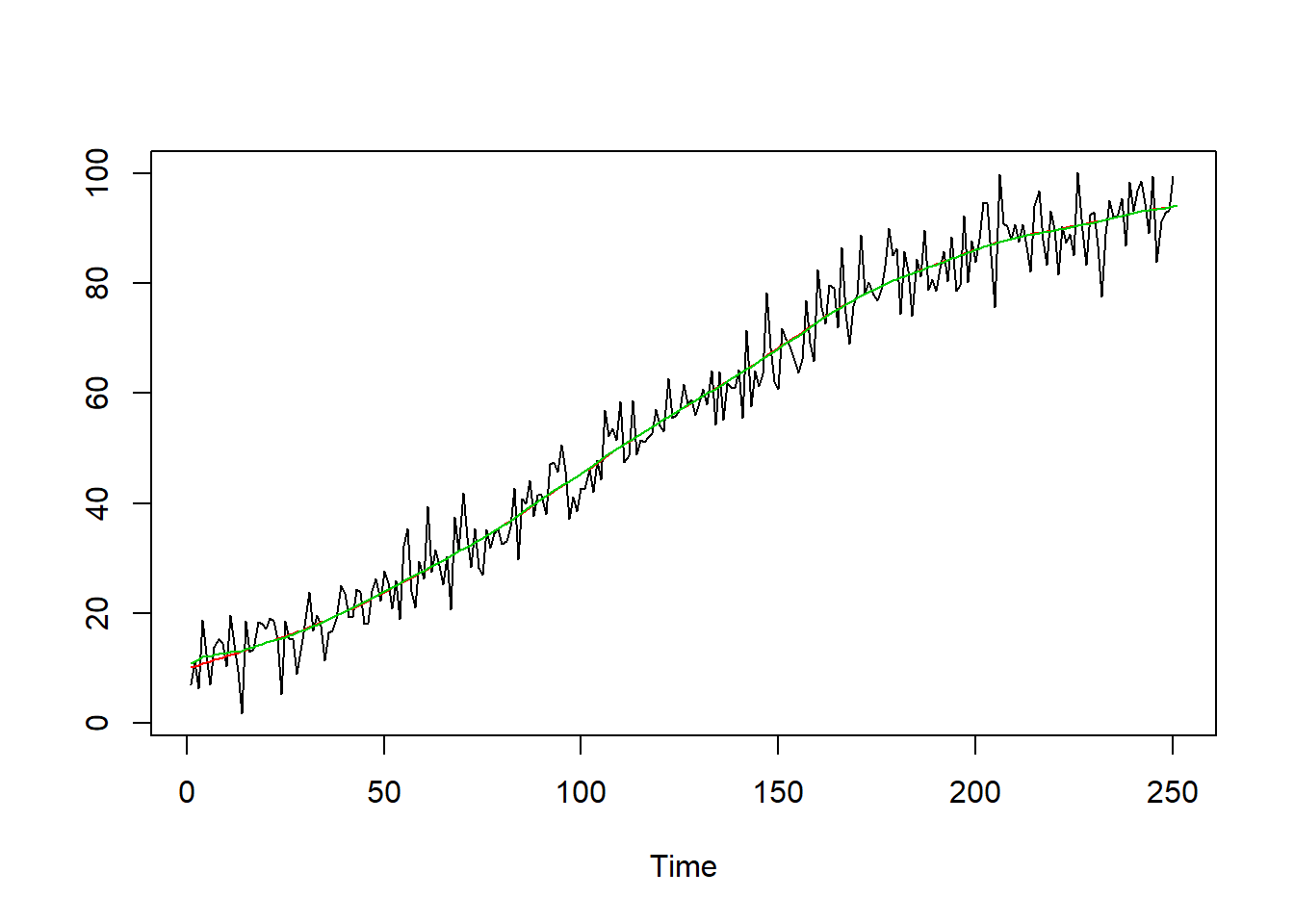Let’s make some predictions based on our MCMC run. We first build a model for future observations, and then call predict method. Note that for EKF based prediction (nsim = 0) the forecasting is based on the summary statistics obtained with EKF, which produces smoother but potentially biased results. future_model <- model future_model$y <- ts(rep(NA, 100), start = end(model$y) + c(1, 0)) out_pred_pm <- predict(out_mcmc_pm, future_model, type = "response", nsim = 50) out_pred_ekf <- predict(out_mcmc_ekf, future_model, type = "response", nsim = 0) library("ggplot2") autoplot(out_pred_pm, y = model$y, plot_median = FALSE)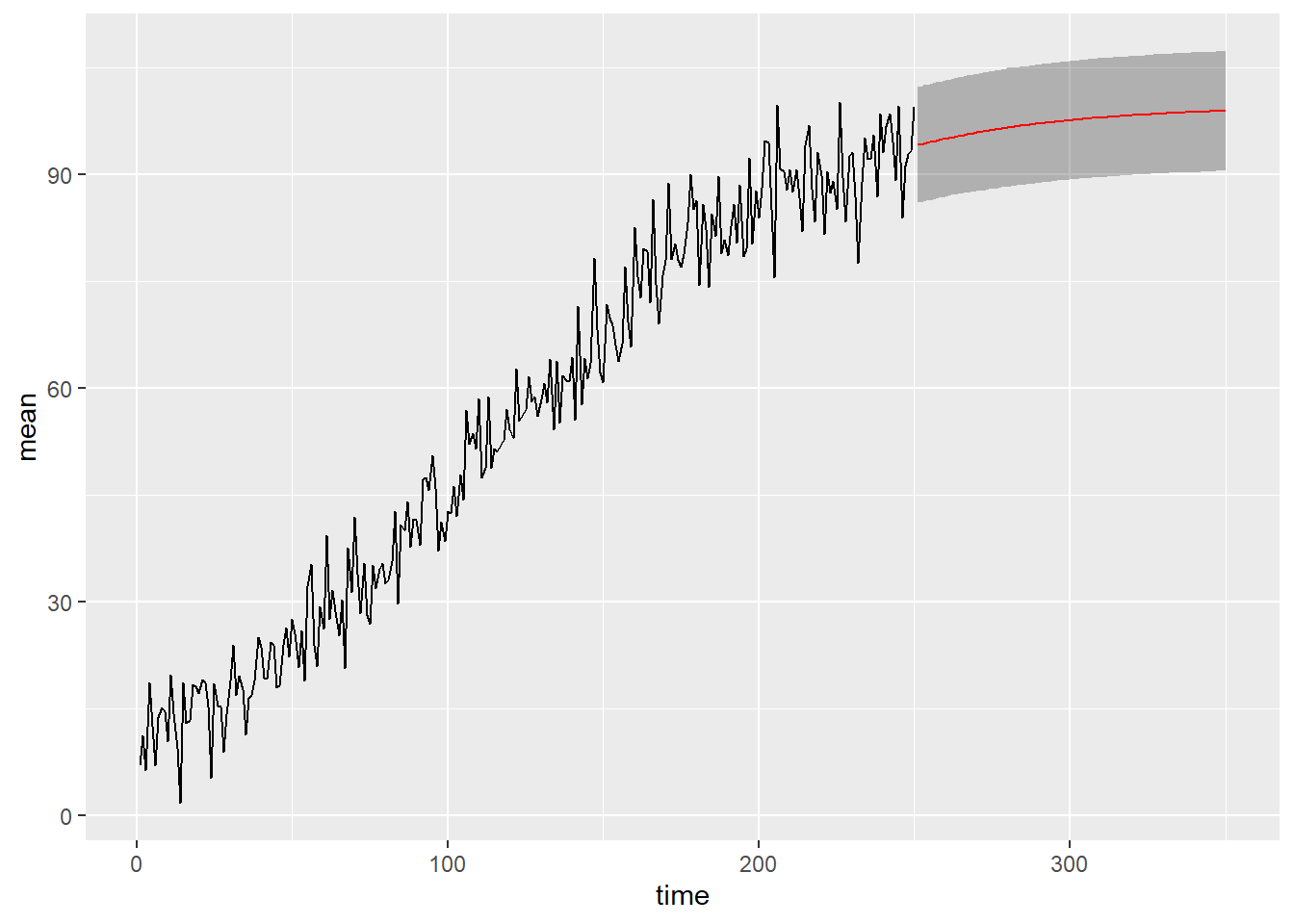autoplot(out_pred_ekf, y = model\$y, plot_median = FALSE)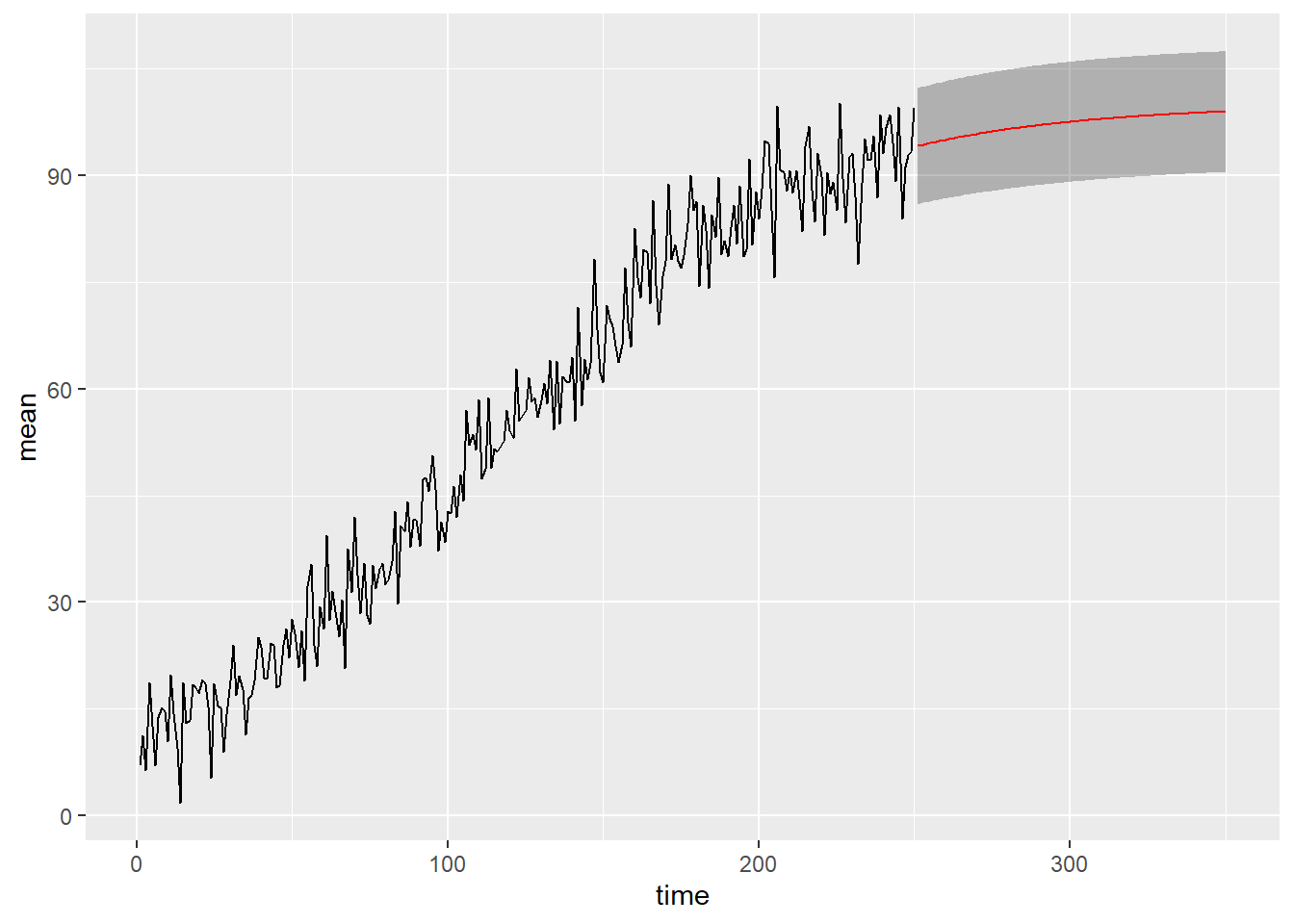## Appendix

This is the full nlg_ssm_template.cpp file:

// A template for building a general non-linear Gaussian state space model
// Here we define an univariate growth model (see vignette growth_model)

// [[Rcpp::interfaces(r, cpp)]]

// Function for the prior mean of alpha_1
// [[Rcpp::export]]
arma::vec a1_fn(const arma::vec& theta, const arma::vec& known_params) {

arma::vec a1(2);
a1(0) = known_params(2);
a1(1) = known_params(3);
return a1;
}
// Function for the prior covariance matrix of alpha_1
// [[Rcpp::export]]
arma::mat P1_fn(const arma::vec& theta, const arma::vec& known_params) {

arma::mat P1(2, 2, arma::fill::zeros);
P1(0,0) = known_params(4);
P1(1,1) = known_params(5);
return P1;
}

// Function for the Cholesky of observational level covariance matrix
// [[Rcpp::export]]
arma::mat H_fn(const unsigned int t, const arma::vec& alpha, const arma::vec& theta,
const arma::vec& known_params, const arma::mat& known_tv_params) {
arma::mat H(1,1);
H(0, 0) = theta(0);
return H;
}

// Function for the Cholesky of state level covariance matrix
// [[Rcpp::export]]
arma::mat R_fn(const unsigned int t, const arma::vec& alpha, const arma::vec& theta,
const arma::vec& known_params, const arma::mat& known_tv_params) {
arma::mat R(2, 2, arma::fill::zeros);
R(0, 0) = theta(1);
R(1, 1) = theta(2);
return R;
}

// Z function
// [[Rcpp::export]]
arma::vec Z_fn(const unsigned int t, const arma::vec& alpha, const arma::vec& theta,
const arma::vec& known_params, const arma::mat& known_tv_params) {
arma::vec tmp(1);
tmp(0) = alpha(1);
return tmp;
}
// Jacobian of Z function
// [[Rcpp::export]]
arma::mat Z_gn(const unsigned int t, const arma::vec& alpha, const arma::vec& theta,
const arma::vec& known_params, const arma::mat& known_tv_params) {
arma::mat Z_gn(1, 2);
Z_gn(0, 0) = 0.0;
Z_gn(0, 1) = 1.0;
return Z_gn;
}

// T function
// [[Rcpp::export]]
arma::vec T_fn(const unsigned int t, const arma::vec& alpha, const arma::vec& theta,
const arma::vec& known_params, const arma::mat& known_tv_params) {

double dT = known_params(0);
double k = known_params(1);

arma::vec alpha_new(2);
alpha_new(0) = alpha(0);
alpha_new(1) = k * alpha(1) * exp(alpha(0) * dT) /
(k + alpha(1) * (exp(alpha(0) * dT) -1));

return alpha_new;
}

// Jacobian of T function
// [[Rcpp::export]]
arma::mat T_gn(const unsigned int t, const arma::vec& alpha, const arma::vec& theta,
const arma::vec& known_params, const arma::mat& known_tv_params) {

double dT = known_params(0);
double k = known_params(1);

double tmp = exp(alpha(0) * dT) /
std::pow(k + alpha(1) * (exp(alpha(0) * dT) - 1), 2);

arma::mat Tg(2, 2);
Tg(0, 0) = 1.0;
Tg(0, 1) = 0;
Tg(1, 0) = k * alpha(1) * dT * (k - alpha(1)) * tmp;
Tg(1, 1) = k * k * tmp;

return Tg;
}

// # log-prior pdf for theta
// [[Rcpp::export]]
double log_prior_pdf(const arma::vec& theta) {

double log_pdf;
if(arma::any(theta < 0)) {
log_pdf = -std::numeric_limits<double>::infinity();
} else {
// weakly informative priors.
// Note that negative values are handled above
log_pdf = R::dnorm(theta(0), 0, 10, 1) + R::dnorm(theta(1), 0, 10, 1) +
R::dnorm(theta(2), 0, 10, 1);
}
return log_pdf;
}

// Create pointers, no need to touch this if
// you don't alter the function names above
// [[Rcpp::export]]
Rcpp::List create_xptrs() {

// typedef for a pointer of nonlinear function of model equation returning vec (T, Z)
typedef arma::vec (*nvec_fnPtr)(const unsigned int t, const arma::vec& alpha,
const arma::vec& theta, const arma::vec& known_params, const arma::mat& known_tv_params);
// typedef for a pointer of nonlinear function returning mat (Tg, Zg, H, R)
typedef arma::mat (*nmat_fnPtr)(const unsigned int t, const arma::vec& alpha,
const arma::vec& theta, const arma::vec& known_params, const arma::mat& known_tv_params);

// typedef for a pointer returning a1
typedef arma::vec (*a1_fnPtr)(const arma::vec& theta, const arma::vec& known_params);
// typedef for a pointer returning P1
typedef arma::mat (*P1_fnPtr)(const arma::vec& theta, const arma::vec& known_params);
// typedef for a pointer of log-prior function
typedef double (*prior_fnPtr)(const arma::vec&);

return Rcpp::List::create(
Rcpp::Named("a1_fn") = Rcpp::XPtr<a1_fnPtr>(new a1_fnPtr(&a1_fn)),
Rcpp::Named("P1_fn") = Rcpp::XPtr<P1_fnPtr>(new P1_fnPtr(&P1_fn)),
Rcpp::Named("Z_fn") = Rcpp::XPtr<nvec_fnPtr>(new nvec_fnPtr(&Z_fn)),
Rcpp::Named("H_fn") = Rcpp::XPtr<nmat_fnPtr>(new nmat_fnPtr(&H_fn)),
Rcpp::Named("T_fn") = Rcpp::XPtr<nvec_fnPtr>(new nvec_fnPtr(&T_fn)),
Rcpp::Named("R_fn") = Rcpp::XPtr<nmat_fnPtr>(new nmat_fnPtr(&R_fn)),
Rcpp::Named("Z_gn") = Rcpp::XPtr<nmat_fnPtr>(new nmat_fnPtr(&Z_gn)),
Rcpp::Named("T_gn") = Rcpp::XPtr<nmat_fnPtr>(new nmat_fnPtr(&T_gn)),
Rcpp::Named("log_prior_pdf") =
Rcpp::XPtr<prior_fnPtr>(new prior_fnPtr(&log_prior_pdf)));

}